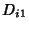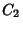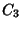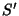## Isodynamic PointsThe first and second isodynamic points of a Trianglecan be constructed by drawing the triangle's Angle Bisectors and Exterior Angle Bisectors. Each pair of bisectors intersects a side of the triangle (or its extension) in two pointsand, for, 2, 3. The three Circles having,, andas Diameters are the Apollonius Circles,, and. The pointsandin which the three Apollonius Circles intersect are the first and second isodynamic points, respectively.andhave Triangle Center Functionsrespectively. The Antipedal Triangles of both points are Equilateral and have Areaswhereis the Brocard Angle.

The isodynamic points are Isogonal Conjugates of the Isogonic Centers. They lie on the Brocard Axis. The distances from either isodynamic point to the Vertices are inversely proportional to the sides. The Pedal Triangle of either isodynamic point is an Equilateral Triangle. An Inversion with either isodynamic point as the Inversion Center transforms the triangle into an Equilateral Triangle.

The Circle which passes through both the isodynamic points and the Centroid of a Triangle is known as the Parry Circle.

See also Apollonius Circles, Brocard Axis, Centroid (Triangle), Isogonic Centers, Parry Circle

References

Gallatly, W. The Modern Geometry of the Triangle, 2nd ed. London: Hodgson, p. 106, 1913.

Johnson, R. A. Modern Geometry: An Elementary Treatise on the Geometry of the Triangle and the Circle. Boston, MA: Houghton Mifflin, pp. 295-297, 1929.

Kimberling, C. Central Points and Central Lines in the Plane of a Triangle.'' Math. Mag. 67, 163-187, 1994.